# Blood Relations and Family Tree - Logical Reasoning (MCQ) Questions

Dear Readers, Welcome to Logical Reasoning Blood Relations and Family Tree questions and answers with explanation. These Blood Relations and Family Tree solved examples with shortcuts and tricks will help you learn and practice for your Placement Test and competitive exams like Bank PO, IBPS PO, SBI PO, RRB PO, RBI Assistant, LIC,SSC, MBA - MAT, XAT, CAT, NMAT, UPSC, NET etc.

After practicing these tricky Blood Relations and Family Tree multiple choice questions, you will be exam ready to deal with any objective type questions.

1. J + K means J is the son of K
2. J - K means J is the wife of K
3. J x K means J is the brother of K
4. J ÷ K means J is the mother of K
5. J = K means J is the sister of K

What does A x B ÷ C mean?

- Published on 15 May 17

a. A is the brother of C
b. A is the father of C
c. A is the uncle of C
d. A is the nephew of C
 Answer  Explanation ANSWER: A is the uncle of C Explanation: A x B ÷ C means A is the brother of B who is the mother of C i.e. A is the uncle of C.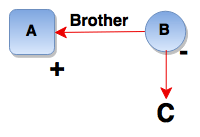1. J + K means J is the son of K
2. J - K means J is the wife of K
3. J x K means J is the brother of K
4. J ÷ K means J is the mother of K
5. J = K means J is the sister of K

What does A + B – C mean?

- Published on 08 May 17

a. C is the father of A
b. C is the uncle of A
c. C is the son of A
d. C is the brother of A
 Answer  Explanation ANSWER: C is the father of A Explanation: A + B – C means A is the son of B who is the wife of C i.e. C is the father of A.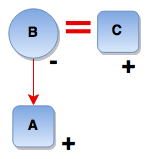1. P x Q means P is the father of Q
2. P - Q means P is the sister of Q
3. P + Q means P is the mother of Q
4. P ÷ Q means P is the brother of Q

Which of the following represents ‘R is niece of M’?

- Published on 08 May 17

a. M – J + R – N
b. R – M x T - W
c. M – K x T - R
d. None of these
 Answer  Explanation ANSWER: M – J + R – N Explanation: R is the niece of M means R is the daughter of brother or sister of M i.e. M – J + R – N or M – J x R – N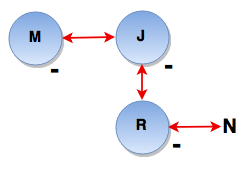1. P x Q means P is the father of Q
2. P - Q means P is the sister of Q
3. P + Q means P is the mother of Q
4. P ÷ Q means P is the brother of Q

Which of the following represents ‘J is the son of F’?

- Published on 08 May 17

a. J ÷ R – T x F
b. J + R – T x F
c. J ÷ M – N x F
d. None of these
 Answer  Explanation ANSWER: None of these Explanation: J ÷ R – T x F means J is the brother of R who is the sister of T who is the father of F i.e. J is the uncle of F. J + R – T x F means J is the mother of R who is the sister of T who is the father of F i.e. J is the grandmother of FJ ÷ M – N x F means J is the brother of M who is the sister of N who is the father of F i.e. J is the uncle of FHere, none of the options follow.

1. P x Q means P is the father of Q
2. P - Q means P is the sister of Q
3. P + Q means P is the mother of Q
4. P ÷ Q means P is the brother of Q

In the expression, B + D x M ÷ N, how is M related to B?

- Published on 08 May 17

a. Son
b. Grandson
c. Granddaughter
d. Daughter
 Answer  Explanation ANSWER: Grandson Explanation: B + D x M ÷ N means B is the mother of D who is the father of M, who, in turn, is the brother of N. Thus, M is the son of D, whose mother is B i.e. M is B’s grandson.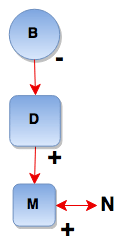1. M \$ N means M is the mother of N
2. M # N means M is the father of N
3. M  @ N means M is the husband of N
4. M % N means M is the daughter of N

If F  @ D % K # H, then how is F related to H?

- Published on 08 May 17

a. Brother-in-law
b. Sister
c. Sister-in-law
d. None of these
 Answer  Explanation ANSWER: Brother-in-law Explanation: F @ D % K # H means F is the husband of D who is the daughter of K who is the father of H i.e. F is the husband of D who is the sister of H i.e. F is H’s brother-in-law.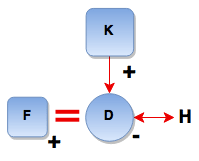1. M \$ N means M is the mother of N
2. M # N means M is the father of N
3. M  @ N means M is the husband of N
4. M % N means M is the daughter of N

If G \$ M @ K, how is K related to G?

- Published on 08 May 17

a. Mother
b. Mother-in-law
c. Daughter
d. Daughter-in-law
 Answer  Explanation ANSWER: Daughter-in-law Explanation: G \$ M @ K means G is the mother of M who is the husband of K i.e. K is the wife of G’s son i.e. K is G’s daughter-in-law.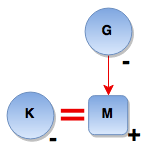1. M \$ N means M is the mother of N
2. M # N means M is the father of N
3. M @ N means M is the husband of N
4. M % N means M is the daughter of N

Which of the following expressions indicate ‘H is the brother of N’?

- Published on 03 May 17

a. H # R \$ D \$ N
b. N % F @ D \$ H
c. N % F @ D % H
d. N % F @ D \$ H # R
 Answer  Explanation ANSWER: N % F @ D \$ H # R Explanation: N % F @ D \$ H # R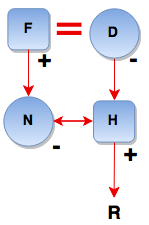1. M \$ N means M is the mother of N
2. M # N means M is the father of N
3. M @ N means M is the husband of N
4. M % N means M is the daughter of N

Which of the following expressions indicate ‘R is the sister of H’?

- Published on 03 May 17

a. H \$ D @ F # R
b. R % D @ F \$ H
c. R \$ D @ F # H
d. None of these
 Answer  Explanation ANSWER: R % D @ F \$ H Explanation: R % D @ F \$ H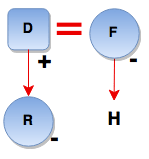1. M \$ N means M is the mother of N
2. M # N means M is the father of N
3. M @ N means M is the husband of N
4. M % N means M is the daughter of N

P  @ Q \$ M # T indicates what relationship of P with T?

- Published on 25 Apr 17

a. Paternal grandmother
b. Paternal grandfather
c. Maternal grandfather
d. Maternal grandmother
 Answer  Explanation ANSWER: Paternal grandfather Explanation: P @ Q \$ M # T means P is the husband of Q who is the mother of M who is the father of T i.e. P is the father of T’s father. P is T’s paternal grandfather.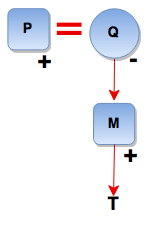1 2 3